# How to Convert Measurements to Inches in Excel

Feb 02, 2020 • edited Feb 03, 2020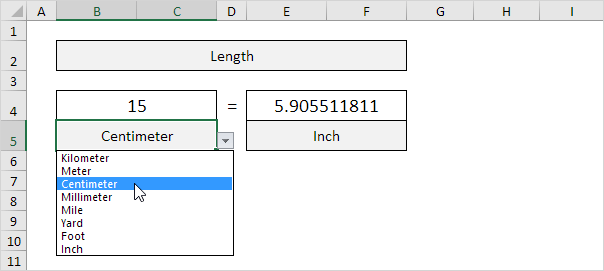Excel offers powerful conversion functionality to the user. You can apply simple equations across ranges of data to quickly produce new figures.

Use this powerful feature to convert centimeters to inches in Excel.

# Cm to inches

1 cm = 0.3937 inch and 1 inch = 2.54 cm. Use a simple formula, the CONVERT function or download our free unit converter to convert from cm to inches or vice versa (see below).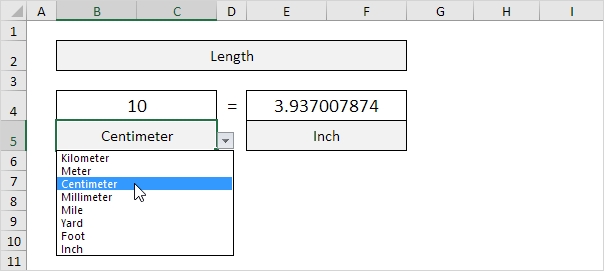1. First, to convert from centimeters to inches, divide by 2.54. The simple formula below does the trick.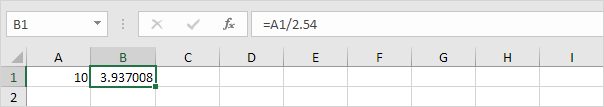2. To convert from inches to centimeters, multiply by 2.54.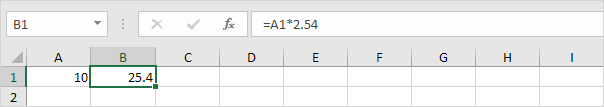3. You can also use the CONVERT function in Excel to convert from centimeters to inches.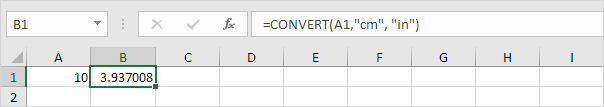Note: the CONVERT function has three arguments (number, from_unit and to_unit).

4. You can also use the CONVERT function in Excel to convert from inches to centimeters.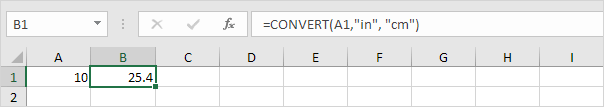Download our free unit converter to quickly and easily convert from cm to inches (or vice versa).

5. Select Length from the category drop-down list.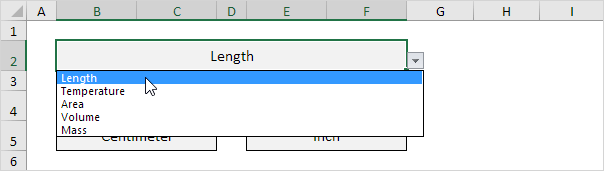Explanation: because you selected Length from this drop-down list, the dependent drop-down lists in cell B5 and cell E5 now contain length units.

6. Enter a number and select the correct length units.Explanation: the unit converter uses worksheet change events to automatically execute VBA code when you change a cell (B2, B4, B5, E4 or E5). The VBA code uses the CONVERT function to convert from one measurement unit to another and uses the VLOOKUP function to lookup the correct unit abbreviations (cm, in, yd, ft, etc.) stored on the second worksheet.

#How To#Tutorial#Formulas #Functions

How To Use Calculate Percentage Discount In Excel

How To Use Not Equal To In Excel